# Second Law of Thermodynamics

Jump to: navigation, search

The Second Law of Thermodynamics is a fundamental truth about the tendency towards disorder in the absence of intelligent intervention. This principle correctly predicts that heat will never flow from a cold body to a warmer one, unless forced to do so by a man-made machine. As the self-described atheist scientist Isaac Asimov admitted:

 “ Another way of stating the second law then is: The universe is constantly getting more disorderly. Viewed that way, we can see the second law all about us. We have to work to straighten a room, but left to itself it becomes a mess again very quickly and very easily. Even if we never enter it, it becomes dusty and musty. How difficult to maintain houses, and machinery, and our bodies in perfect working order: how easy to let them deteriorate. In fact, all we have to do is nothing, and everything deteriorates, collapses, breaks down, wears out, all by itself -- and that is what the second law is all about. ” —Isaac Asimov, Smithsonian Institute Journal, June 1970, p. 6, emphasis added

The Second Law of Thermodynamics is the result of the intrinsic uncertainty in nature, manifest in quantum mechanics, which is overcome only by intelligent intervention. As explained in the Hebrews 1:10, the universe shall "wear out" like a "garment", i.e., entropy is always increasing.

This law makes it impossible to build a perpetual motion machine - the increase in entropy inevitably derails the system even if energy remains constant. This law also predicts the tendency for cables, cords, and wires, to self-entangle and repeatedly require straightening.

The Second Law of Thermodynamics disproves the atheistic Theory of Evolution and Theory of Relativity, both of which deny a fundamental uncertainty to the physical world that leads to increasing disorder.

In the terminology of physics, the Second Law states that the entropy of an isolated or closed system never decreases.

## Entropy and disorder

In this context "increasing disorder" means the decline in organization that occurs without intelligent intervention. Imagine your old room at your parent's house. Remember how easy it was to let the room turn into a uniform mess (disorder) and remember how hard it was to clean it up until it fit a specific, non-uniform design (order). Not cleaning up would always result in an increase of entropy in your room!

The flow of energy (by heat exchange) to places with lower concentrations is called the "heat flow."

The often-heard argument that this law disproves an eternal universe is true, because in that case maximum entropy would have been reached already. A counterargument to this would be to suggest that the universe is still in the process of approaching maximum entropy.

There are many different ways of stating the Second Law of thermodynamics. An alternative statement of the law is that heat will tend not to flow from a cold body to a warmer one without intelligent intervention, or work, being done, as in the case of a refrigerator. Other statements include that it is impossible for an engine to convert heat perfectly (I.e. at 100% efficiency) into work. These statements are qualitative and stating the Second Law in terms of entropy makes the law quantitative.

This law makes it impossible to build a perpetual motion machine - the increase in entropy inevitably derails the system even if its energy remains constant (as described by the first law of thermodynamics).

The Second Law can be expressed mathematically as: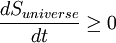whereis the rate of change of entropy of the universe with respect to time.

Another way of viewing the Second Law is in terms of probability. In nature there is no one to clean up the universe, only chances. The chance of something becoming orderly is essentially zero, which it is a certainty that things will become more disorderly.

On a universal scale a tidy room would be a universe which has pockets of above average concentrations of energy (if you - incorrectly - assume relativity E=mc² this includes matter as well.)

## Second Law compared with other physical laws

Thermodynamics occupies an unusual place in the world of science, particularly at the high school and undergraduate levels. The Second Law is the one that is especially peculiar. (In fact, the other laws are comparatively mundane. The first law is just a statement that heat is a form of energy, and that energy, whether in the form of heat or not, is conserved. This was a very nontrivial result at first, but, with the understanding of heat and temperature that later developed, it's quite unremarkable. The third law is a statement that absolute zero can't be reached by any finite number of Carnot cycles. While true, its significance pales in comparison to that of the Second Law.)

Perhaps what makes the Second Law so remarkable is that it describes irreversible phenomena. In particular, it describes the observed fact that heat energy, in bodies that are not being externally manipulated by compression, etc., flows only from a warmer body to a cooler one. When a warmer body is placed in contact with a cooler one, heat energy will flow (always preserving total energy, of course) from the warmer one to the cooler one. The warmer one will cool off as it releases its energy, and the cooler one will warm up. This process will continue until the two bodies reach the same temperature, or "thermal equilibrium".

Until the development of statistical mechanics, no one knew why this was so, or what temperature actually meant. What was known was simply that a body with a higher temperature would send heat to a body with a lower temperature, no matter what the bodies were made of.

The fact that this kind of heat flow is irreversible makes the whole field of thermodyamics lie outside of the realm of classical Newtonian mechanics or Relativistic mechanics. In Newtonian or Relativistic mechanics, every phenomenon can go in reverse order. The catchy phrase Arrow of time (or "time's arrow") was coined by Arthur Eddington to denote this one-way behavior not shared by other theories of physics.

The field of statistical mechanics attributes the increase in entropy to the statistical tendencies of huge aggregates of particles at the molecular or atomic level. While Newtonian and Relativistic mechanics can, in principle, precisely describe assemblages of any number of particles, in practice they are not directly applied to the behavior of bulk material. That is, they are not applied to a number of particles on the order of Avogadro's number.

Statistical mechanics makes no assumptions about the microscopic cause of the seemingly random behavior. Statistical mechanics was developed in the 19th century prior to quantum mechanics, and does not depend on the Heisenberg uncertainty principle. The quantum mechanical uncertainty goes away at the microscopic level once the system is observed and the wave function collapses.

It needs to be emphasized that the peculiarities of thermodynamics, with the phenomenon fo irreversibility and the "arrow of time", in no way contradict the (reversible) processes of classical Galilean, Newtonian, Lagrangian, or Hamiltonian physics, or of special or general relativity. Those formulations are precise in the regimes in which the behavior of individual particles are analyzed. When two gas molecules collide in the Kinetic theory, that collision is perfectly reversible. It is only when one goes into a problem domain in which the bulk behavior of huge numbers (on the order of Avogadro's number) of particles are analyzed, without regard for the individual particles, that thermodynamics and statistical mechanics come into play.

## Elementary probability and statistics

If you flipped a coin 20 times and it came up heads each time, you would consider that to be a remarkable occurrence. (Perhaps so much so that you would inspect the coin to be sure it didn't have heads on both sides.) If you tried it and got tttthtththhhththhthh, you would probably not consider it remarkable. Yet each of these outcomes is equally probable: about 1 in 106. If you shuffled a deck of cards and found them all in exact order from the 2 of clubs to the ace of spades, you would consider that to be very remarkable. But if you got the distribution shown in the illustration on page 314 of Alfred Sheinwold's 5 Weeks to Winning Bridge, you would probably consider it just "random". Yet each of these orderings has the same probability of occurring—1 in 52 factorial, which is about 1066.

For small sets such as coin tosses or card shufflings, what constitutes "random" vs. "well ordered" is in the eye of the beholder. If a room in your house started in a state that most people would consider "neat and tidy", and you went into that room every day, picked up a random obect, and threw it against a random wall, after a month most people would consider the room a mess. But, once again, this is hard to quantify. The kinds of statistical analyses that are required for the study of thermodynamics have to be much more careful than this. The kind of folksy quotations in popular articles about messy rooms, as in the Isaac Asimov quote, may sell magazines, but they don't shed much light on how thermodynamics works.

What is needed is an analysis of aggregate properties, not individual items. We need to quantify the results. For the case of the coin toss, we might ask how many times we got heads. The probabilities can be worked out; they are a "Gaussian distribution", also known as a "bell curve". The probability of heads 0 times out of 20 is 1 in 1048576. Getting heads exactly 1 time is .00002, and so on, as shown in this table. Notice that getting heads exactly 10 times is the most probable outcome, but its probability is still only 18%. If we did the experiment a larger number of times, the probability of exactly 50% heads would still be higher than any other, but it would be quite small. What is important is the probability of getting a certain number of heads or less.

Number of times it

comes up heads

Probability of that

exact number

Probability of that

number or less

0 10-6 10-6
1 .00002 .00002
2 .00002 .0002
3 .0011 .0013
4 .0046 .0059
5 .0148 .0207
6 .0370 .0577
7 .0739 .1316
8 .1201 .2517
9 .1602 .4119
10 .1762 .5881
11 .1602 .7483
12 .1201 .8684
13 .0739 .9423
14 .0370 .9793
15 .0148 .9941
16 .0046 .9987
17 .0011 .9998
18 .00002 .99998
19 .00002 .99999
20 10-6 1.000

With really large numbers, the probability of any particular outcome is vanishingly small; the only sensible measure is the accumulated probability, or the probability density, measured in a way that doesn't involve individual outcomes.

## Extreme probability and statistics

The Second Law of Thermodynamics derives from this fundamental principle:

The properties of an aggregate of measurements, when the individual measurements are not predetermined, tend toward the "most probable" distribution.

The measurements could be things like whether a coin came up heads, whether a card in a deck has a certain value, or the energy of a gas molecule. The fundamental truth of this, for reasonable numbers of things like coins or cards, is quite sensible on the intuitive level. These principles were worked out, by Fermat and others, in the 17th and 18th century. The same principles apply when the numbers are enormous, on the order of Avogadro's number, but some intuitive conclusions can be misleading.

When dealing with thermodynamics, we are dealing with the statistical aggregate behavior of macroscopic pieces of matter, so we have to increase the number of items from 10, or 52, to something like Avogadro's number. So the number of possible situations, instead of being 106 or 1066, is something like 10Avogadro's number, that is, 101023. The enormity of such a number makes a huge amount of difference. If you flip a coin Avogadro's number of times, it will come up heads about half the time, as before. But, for all practical purposes, we can say that it will come up heads exactly half the time. The number of heads might be off by a few quintillion (this is the "law of large numbers"), but that won't make any practical difference.

• You can't ask any questions about individual items—air molecules don't have labels like "Jack of Diamonds". You can only ask questions about the aggregate behavior of macroscopic pieces of space.
• While the probabilities of certain outcomes can be mathematically calculated, they are so small that, as a practical matter, we can say that they do not occur. People sometimes like to say things like "The Second Law of Thermodynamics means that it is very unlikely that heat will travel from a colder object to a warmer one." That's a fallacious way of thinking about it. It is a statistical impossibility—it just doesn't occur.
• An example is the question of how likely it is that all the air molecules in a room will move to one corner, asphyxiating everyone. This is sometimes worked out in physics classes. But the conclusion has to be that this occurrence, or anything remotely resembling it, might have a probability on the order of 1 in 101023—it just doesn't happen.

## Aren't we cheating when we say that heat flows from a warmer body to a colder one, when all we really know is that it "probably" flows that way?

Well, yes, and no. The laws of probability and statistical mechanics are precisely true, since they are mathematical theorems. They are as precise as that 2+2=4 exactly. It's their application to the real world of molecules and such that is merely "probably" correct. When we say that heat flows downward in macroscopic objects (ice cubes, teapots, Carnot engines), what we really mean is that it flows downward with a probability of 99.999<put in Avogadro's number of 9's here>999 percent. Everyone, scientists and lay people alike, accept this as true. It is the basis for just about everything, including breathing. No one ever questions the reading on the pressure gauge of a gas canister on the grounds that it is only probably correct, or is surprised when one blows into a balloon and it expands.

So the fine philosophical point about it being only probably correct is just that—a fine philosophical point.

## So is the second law falsifiable?

Absolutely. The laws of statistics and probability are mathematical theorems and are absolutely true. The only thing for which falsifiability is an interesting question is the application of those theorems to the real world. Statements like "if you flip a coin 10 times it will never come up heads each time" is both falsifiable and false. One can easily imagine an experiment that would refute it—flip the coin and have it come up heads 10 times. One could even do the experiment, getting that result, if one had the patience for it.

But when we go from 10 coins to macroscopic objects like ice cubes or teapots, one can still imagine an experiment. If all the air molecules in one's house spontaneously moved to the East wall or the West wall, the pressure on those walls would make the house blow up, probably taking several nearby houses with it. So an observation of this phenomenon would refute the second law, whether it's ever been observed or not. (It hasn't.)

## Application to molecular behavior

Statistical mechanics is the application of probability to enormous numbers like this. It was developed, along with the kinetic theory, by James Clerk Maxwell, Ludwig Boltzmann, Rudolf Clausius, Benoît Paul Émile Clapeyron, and others, during the 19th century. The development of the kinetic theory of gases, statistical mechanics, and thermodynamics revolutionized 19th century physics. It was recognized that, while we can't analyze the behavior of every molecule, we can analyze the statistical behavior of macroscopic assemblages. When gas molecules collide, they can transfer energy in a manner that leads to the principle of equipartition of energy. This, plus the constraints on conservation of the total energy, leads to the Maxwell-Boltzmann distribution of molecular energies. From this, one can deduce the properties of volume, pressure, and temperature, leading to Boyle's law and Charles' law, among others. Temperature was found to be just the average energy per molecule. (Actually, the average energy per "degree of freedom".)

The fact that heat only flows downhill, and that entropy never decreases, is now just a consequence of the "most probable distribution" principle, or equipartition principle, from mathematical statistics, albeit at a vastly larger scale.

### Example of the Statistical nature of the Second Law

To see this divide a container two and suppose that 1 half contains 20 molecules and the other none. What we expect to happen is for the molecules to spread out and have roughly half (10) in each half of the container. We can calculate the number of micro-states,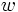, which correspond to each macro-state:

Macro-state Number of Micro-states,Entropy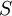All particles in one half 1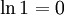1 in left and 19 in right 20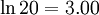10 in left and 10 in right 184756Note that the Boltzmann constant has taken a value of 1 to simplify the maths. So the state that we would expect to find the system in, the last one, has the highest entropy. However, the system could be in this state (10 in left, 10 in the right) and, just by chance, all the molecules could make their way to the left hand side of the box. This corresponds to a decrease of entropy. This example could be expanded up to a room, so why do we never see all the air in a room suddenly move to one end? The reason is that it is so unlikely, perhaps less than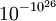, that it essentially never occurs. Hence it may be assumed that for most systems entropy never decreases. This is known as the fluctuation theorem.

## Thermodynamic definition of entropy

One of the things that came out of the development of statistical mechanics was the definition of entropy as a differentialS = entropy, Q = heat energy, T = temperature

The definition as a differential implies that it can be considered to be independent up to an additive constant. This is true; for problems in thermodynamics it doesn't matter whether a constant is added to the entropy everywhere. (But this is not true for the definitions used in combinatorics and mathematical statistics.)

The definition as a differential could mislead one into thinking that integration around some closed path could lead to a different value, that is, that dS is not an exact form. This is not correct—dS is an exact form, and entropy is a true state variable. A mole of uniform Nitrogen at standard temperature and pressure (STP) always has the same entropy, once one chooses the additive constant for the system. (It is; see below.) A mole of nitrogen that is partly at one temperature and partly at another will have a lower entropy; the entropy will increase as the gas fractions mix.

The measure of entropy of a specific thing is in Joules per Kelvin, as can be seen from the definition as dQ/T. Entropy is an "extensive" quantity' like energy or momentum—two teapots have twice the entropy of one teapot. So the entropy of some kind of substance (e.g. nitrogen at STP) could be measured in Joules per (mole Kelvin). This happens to be the same dimensions as the universal gas constant R.

## Entropy in popular culture—intuitive notions of entropy and "randomness"

The tendency toward disorder is something that is accessible on an intuitive level—shuffling a deck of cards destroys their order, rooms don't clean themselves up, and so on. This phenomenon is often described by "popular science" writers, such as the Isaac Asimov quote at the beginning of this page. As a further example of this, a Google search for "entropy" yields "lack of order or predictability; gradual decline into disorder", with synonyms like "deterioration" and "degeneration".

This is all very true, because the underlying phenomena of mathematical statistics are the same, but the difference in scale makes an enormous difference, and this can be misleading if one tries to draw thermodynamical conclusions from this intuitive notion.

When one bridges the gap between thermodynamics and the intuitive notions of entropy from ordinary statistics, one comes up with the statistical definition of entropy: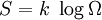where k is Boltzmann's constant of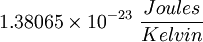and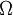is the number of possible configurations of whatever is being analyzed. Boltzmann's constant is in Joules per Kelvin rather than Joules per (mole Kelvin), because the mole has been replaced with the individual molecule.

The equivalence of the thermodynamical and statistical definitions of entropy is derived by statistical mechanics, and, in particular, the principle of equipartition of energy.

For a mole of an ideal gas undergoing a temperature change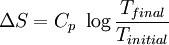whereis the heat capacity at constant pressure. This can be integrated to getFor a diatomic ideal gas such as Nitrogen,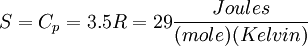soPeople are generally accustomed to how probabilities work in everyday life. Poker players know what the odds are that a hand is a full house or a straight flush, and Blackjack players know the odds of going over 21 on the next card. They have a feel for the shape of the bell curve. But when the sample size is what it is in thermodynamics, this intuition fails completely—the bell curve becomes incredibly sharp and high. The odds of getting heads 1/4 of the time or less on 20 coin tosses is, as noted above, 2.07%. But if the coin is tossed Avogadro's number of times (6.022 x 1023), it will, with virtual certainty, come up heads 3.011 x 1023 times, give or take a few trillion. Any measurable deviation is a statistical impossibility.

Using the definition of entropy from mathematical statistics, the entropy of a completely random deck of cards is K log 52!, which is 2.16 x 10-21 Joules per Kelvin. The entropy of a sorted deck is zero.

This misleading nature of statistics at the thermodynamical level can lead to some misconceptions. As an example, the entropy effects of shuffling or sorting a deck of cards are utterly insignificant compared with the effects of thermodynamic entropy. A mole of Nitrogen (22.4 L, 28 g) at 20 degrees Celsius, containing a random deck of cards, has the same entropy as a mole containing a completely sorted deck at 20.0000000000000000000015 degrees Celsius. One can easily be misled if one ascribes macroscopic visible phenomena of apparent randomness to the Second Law of Thermodynamics.

The temptation is to let one's perception of "disorder", or "messiness", or "degradation", as in the Isaac Asimov quote given above, or the result of Googling "entropy", color one's views of the Second Law. The Second Law says very specific things about heat flow. Perceptions of "disorder" generally do not involve heat flowing from a warmer body to a cooler one.

As an example, a person sorting a deck of cards, or cleaning up their room, of course needs some external source of low entropy in order not to violate the Second Law. That entropy reduction effect is easily provided by the food one eats and the air one breathes. The "entropy budget" required to sort a deck of cards can be provided by the metabolism of about 10-29 grams of sugar. Of course a human metabolizes vastly more food energy during that time.

As another example, the devastation of a forest fire could be thought of as leading to a less ordered state. But in fact the entropy of the ashes is probably lower than that of the trees, because energy is released during the combustion. In any case, the forest grows back. Where does the "entropy budget" come from? Sunlight.

As another example, an apple left lying around will "deteriorate". But it is also true that apple trees turn dirt into apples. Once again, the entropy budget comes from sunlight.

In fact, entropy changes locally all the time, on a vastly larger scale than cards, rooms, apples, people, or fires—higher during the day, lower at night, higher in Summer, lower in Winter. Entropy is constantly being "wasted". Where does the entropy reduction required to offset this come from? The Earth is a giant heat engine, with energy from the Sun falling on the daylight side, and "waste" energy emitted into space from the night side. This sunlight powers virtually everything that happens on Earth.

## Entropy and information

Information is in the eye of the beholder. The first ten million digits of pi can be considered to be a very precise and detailed piece of information, or they can be considered to be ten million digits of random garbage. In the former case, the digits have an entropy of zero; in the latter case the entropy is 3.2 x 10−21 Joules per Kelvin.

The entropy of the human genome, in each cell, is 6 x 10-14 Joules per Kelvin when the base pairs are considered to be random, and zero when the base pairs are in a given human's specific genetic configuration. The metabolism of 2.5 x 10-22 grams of sugar, or one nanosecond of sunlight falling on one square centimeter, can provide the "entropy budget" to copy a cell's genome. That is, it can turn the random base pairs floating in the intracellular fluid into a copy of an existing set of chromosomes. This is done every time a cell divides. Of course the process is far from 100% efficient. 10-8 grams of sugar, or 4 seconds of sunlight falling on one square meter, are sufficient, under 100% thermodynamic efficiency, to copy the DNA of all the cells in one's body. Avogadro's number really is very large.

## Reversibility and irreversibility

Reversibility is a theoretical concept related to the Second Law of Thermodynamics. A process is reversible if the net heat and work exchange between the system and the surroundings is zero for the process running forwards and in reverse. This means the process does not generate entropy. In reality, no process is completely reversible. Irreversibility is a quantity sometimes called "lost work" and is equal to the difference between a process' actual work and reversible work. Irreversibility is also equal to a process' entropy generation multiplied by a reference temperature.

## Trend toward uniformity in the universe

The universe will always become increasingly uniform, that is: heat will spread until the entire universe has the temperature and energy level (in an isolated system heat will always spread from a place where there is a lot of heat to a place where there is less until balance is achieved), forces will continue to work until a universal balance has been achieved.

In this final state the universe is one uniform space where nothing happens and no work (moving something) can be done since there are no above average concentrations of energy left. This state is called maximum entropy and is said to be in perfect disorder (although intuitively its uniformity would seem to be a state of perfect order) because it has become impossible to determine what happened in the past. i.e. There are an infinite number of ways (histories of the universe) maximum entropy could have been reached.

## The types of systems governed by the Law

The Second Law of Thermodynamics is essentially a conservation law, like conservation of energy, or momentum, or mass. As such, it only applies to isolated systems. One would not perform an experiment to show conservation of energy in a swinging clock pendulum if one pushes on the pendulum, and one can't show conservation of momentum of a baseball at the instant that it is hit by a bat. By the same token, the Second Law can't be trusted when applied to a system that heat energy is entering or leaving. Adding or removing matter would be an even more serious failure.

There is only one type of system that the Second Law of Thermodynamics applies to: an isolated system. An isolated system is one that does not exchange matter or energy with its surroundings.

Hence the Second Law of Thermodynamics does not strictly apply to the following types of system:

• Closed system - Exchanges energy, but not matter, with its surroundings
• Open system - Exchanges both matter and energy with its surroundings

The boundary of any system can always be expanded to produce an isolated system, even if that system has to be the entire universe.

## Liberal abuse of the Second Law of Thermodynamics

The Second Law of Thermodynamics is abused by many people, claiming that it buttresses their arguments for a wide variety of things to which it simply doesn't apply. It has become common in recent years for environmentalists to claim that the Second Law of Thermodynamics implies limits to economic growth. Their reasoning is that because free energy in resources such as oil decreases with time, then economic growth can only be finite. (The supply of oil is finite, but the consequences of that are not consequences of the Second Law.) However, this simplistic liberal reasoning ignores the non-zero sum nature of free market economics, whereby improvements in technology deliver gains for all at no further cost. Indeed, one of the most vital economic goods, knowledge, or more generally information, can be said to be free from thermodynamic limitations entirely. Liberals also vastly exaggerate the limitations that natural resources impose on human economies. Some estimate that the Earth can harbor 100 billion people. God Himself gives His explicit assurance that the Earth will be generous as long as the human race exists in Genesis: "And God blessed them, and God said unto them, Be fruitful, and multiply, and replenish the earth, and subdue it: and have dominion over the fish of the sea, and over the fowl of the air, and over every living thing that moveth upon the earth." (Gen. 1:28, KJV)

## Creation Ministries International on the Second Law of Thermodynamics and evolution

The Second Law of Thermodynamics attracts a lot of attention in religious websites and books. Creation Ministries International has a great wealth of information on why the Second Law of Thermodynamics is incompatible with the evolutionary paradigm.

Some of their key resources on this matter are:

 “ I tend not to use entropy arguments at all for biological systems. I have yet to see the calculations involving either heat transfer or Boltzmann microstates involved for natural selection. Until creationists can do that, they should refrain from claiming that organic evolution contradicts the Second Law; a trite appeal to “things become more disordered according to the Second Law” is inadequate. ” —Jonathan Sarfati, in the cited work

The main argument against evolution using the second law of thermodynamics is that evolution requires a decrease in entropy (disorder). However, the second law of thermodynamics states that entropy increases, so the two are contradictory. These resources misrepresent the Second Law of Thermodynamics, ignoring the fact the earth is not an isolated system (energy is added from the sun for example).

## The 1st and 2nd law of thermodynamics and the universe having a beginning

According to Ohio State University professor Patrick Woodward, the First Law of Thermodynamics "simply states that energy can be neither created nor destroyed (conservation of energy)."

The Christian apologetics website Why believe in God? declares about the Second Law of Thermodynamics:

 “ The second law of thermodynamics, or the law of increased entropy, says that over time, everything breaks down and tends towards disorder - entropy! Entropy is the amount of UNusable energy in any systems; that system could be the earth's environment or the universe itself. The more entropy there is, the more disorganisation and chaos. Therefore, if no outside force is adding energy to an isolated system to help renew it, it will eventually burn out (heat death). This can be applied to a sun as well as a cup of tea - left to themselves, both will grow cold. You can heat up a cold tea, you cannot heat up a cold sun. NOTE: when a hot tea in an air tight room goes cold (loses all it's energy) not only do we NOT expect the process to reverse by natural causes (ie. the tea will get hot again), but both room temp and tea temp will be equal. Keep that in mind as you read the next paragraph. Look at it like this, because the energy in the universe is finite and no new energy is being added to it (1st law), and because the energy is being used up (2nd law), the universe cannot be infinite. If our universe was infinite but was using up a finite supply of energy, it would have suffered 'heat death' a long time ago! If the universe was infinite all radioactive atoms would have decayed and the universe would be the same temperature with no hot spots, no bright burning stars. Since this is not true, the universe must have begun a finite time ago. ”

Thus, the First Law of Thermodynamics and the Second Law of Thermodynamics suggests that the universe had a beginning. This argument is similar to the argument for the big bang, that the universe is expanding now, so in the past it must have been smaller, and since there is a limit to how small the universe can be, it points to a beginning. In this argument, it is entropy that has a lower limit.

However, the statistical nature of the second law mean that it is not firmly true. If the universe is infinite in time, then eventually all possibilities, no matter how small, are played out. In this way, the universe could reach maximum entropy and then happen, by chance, to return to a low entropy state. This would happen an infinite number of times. Hence we could be in the process of entropy increasing and the universe need nor have a beginning.

## The 1st and 2nd laws of thermodynamics, theism and the origins of the universe

In the articles below, theists point out that the First Law of Thermodynamics and the Second Law of Thermodynamics point to the universe having a divine origin: